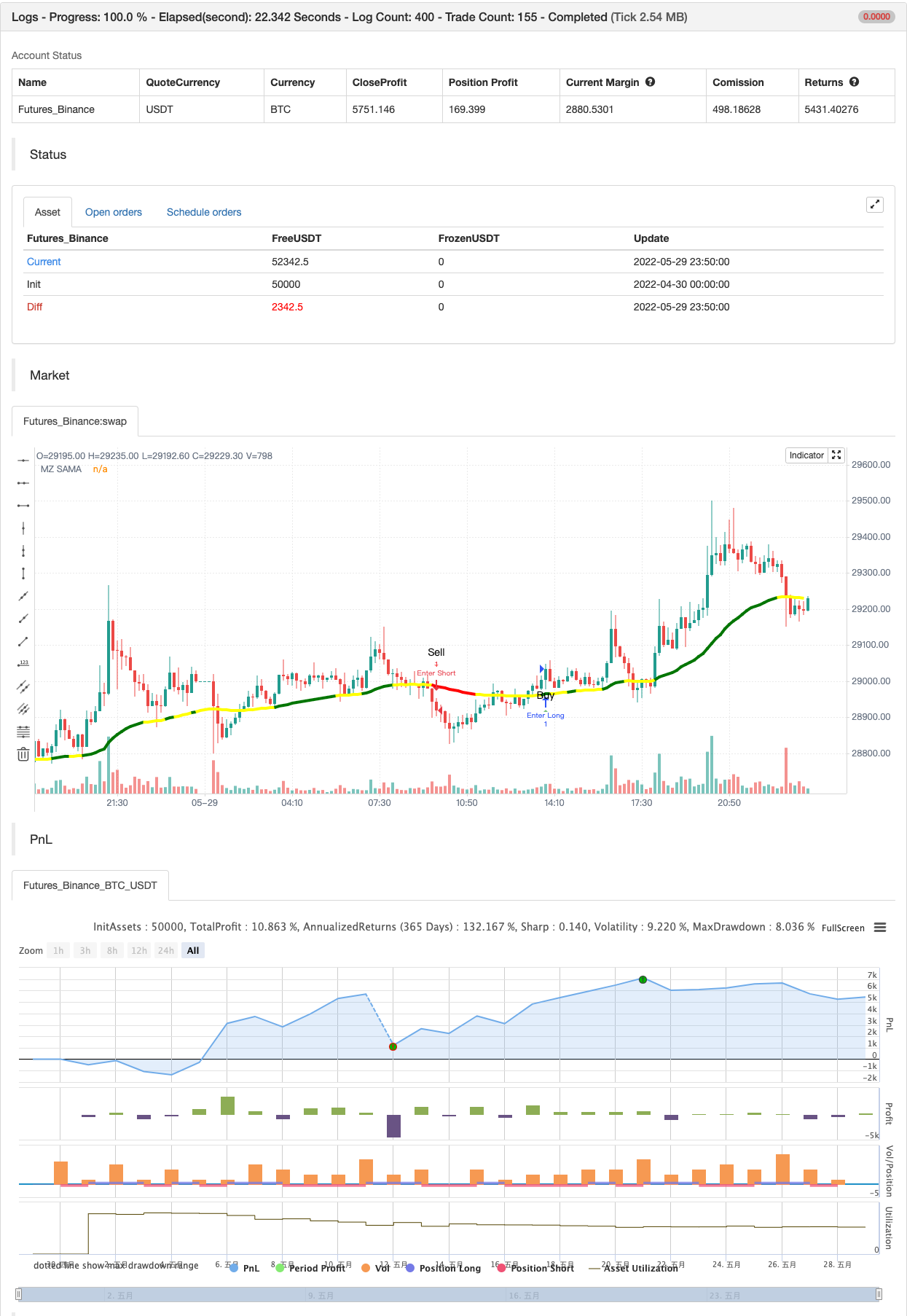Author: 张超, Date: 2022-05-31 18:07:34
Tags: AMA

INTRODUCTION

This script is inspired from “Vitali Apirine (Stocks & Commodities V.36:5: Adaptive Moving Averages)” and a correction to Dynamic Volume Adaptive Moving Average (MZ DVAMA). I have used slope filtering in order to adapt trends more precisely for better trades. Slope adaption makes it better for adaptive moving average to detect trend health; making it easier to make decisions based on market strong price momentums, consolidations or breakouts. This isn’t possible with only using simply Adaptive Moving Averages. Adaptive Moving Averages curve doesn’t change its length based on Slope but it uses slope adaptive color for trend strength detection.

TREND DETECTION Green Color: Strong Uptrend with good price momentum. Red Color: Strong Downtrend. Yellow Color: Market is either choppy, sideways or consolidating. Better to avoid taking new positions and if trade is running then its good to carry it on.

DEFAULTS SETTINGS AMA length is 200 (Better for timeframes higher than 1H) Minor length is 6 Major length is 14 Slope period is set to 34 with 25 of initial range. Consolidation is always below 17. ALERTS Buy/Sell Alerts will follow on when slope is out of consolidation/ choppiness area. Best entry is at absolute alerts timing but other trades can be started midway based on trend condition.

backtest```/*backtest
start: 2022-04-30 00:00:00
end: 2022-05-29 23:59:00
period: 10m
basePeriod: 1m
exchanges: [{"eid":"Futures_Binance","currency":"BTC_USDT"}]
*/

// This source code is subject to the terms of the Mozilla Public License 2.0 at https://mozilla.org/MPL/2.0/

//@version=5

indicator('Slope Adaptive Moving Average (MZ SAMA)', shorttitle='MZ SAMA', overlay=true)

/////////////////////////////////////////////////////////////////////
/////////////////////////////////////////////////////////////////////
/////                        MZ SAMA                           //////
/////////////////////////////////////////////////////////////////////
/////////////////////////////////////////////////////////////////////

chartResolution = input.timeframe('', title='Chart Resolution')
src = input.source(close, 'Source')

// Length Inputs
string grp_1 = 'SAMA Length Inputs'
length = input(200, title='Adaptive MA Length', group = grp_1) // To check for Highest and Lowest value within provided period
majLength = input(14, title='Major Length', group = grp_1)     // For Major alpha calculations to detect recent price changes
minLength = input(6, title='Minor Length', group = grp_1)      // For Minor alpha calculations to detect recent price changes

// Slope Inputs
string grp_2 = 'Slope and Dynamic Coloring Parameters'
slopePeriod = input.int(34, title='Slope Period', group = grp_2)
slopeInRange = input.int(25, title='Slope Initial Range', group = grp_2)
flat = input.int(17, title='Consolidation area is when slope below:', group = grp_2)
bull_col = input.color(color.green, 'Bull Color  ', inline='dyn_col', group = grp_2)
bear_col = input.color(color.red, 'Bear Color  ', inline='dyn_col', group = grp_2)
conc_col = input.color(color.yellow, 'Reversal/Consolidation/Choppiness Color  ', inline='dyn_col', group = grp_2)

showSignals = input.bool(true, title='Show Signals on Chart', group='Plot Parameters')

//Slope calculation Function to check trend strength i.e. consolidating, choppy, or near reversal

calcslope(_ma, src, slope_period, range_1) =>
pi = math.atan(1) * 4
highestHigh = ta.highest(slope_period)
lowestLow = ta.lowest(slope_period)
slope_range = range_1 / (highestHigh - lowestLow) * lowestLow
dt = (_ma - _ma) / src * slope_range
c = math.sqrt(1 + dt * dt)
xAngle = math.round(180 * math.acos(1 / c) / pi)
maAngle = dt > 0 ? -xAngle : xAngle
maAngle

//MA coloring function to mark market dynamics

dynColor(_flat, slp, col_1, col_2, col_r) =>
var col = color.new(na,0)
// Slope supporting bullish uprtrend color
col := slp > _flat ? col_1:
// Slope supporting bearish downtrend color
slp <= -_flat ? col_2:
// Reversal/Consolidation/Choppiness color
slp <= _flat and slp > -_flat ? col_r : col_r
col

//AMA Calculations

ama(src,length,minLength,majLength)=>
minAlpha = 2 / (minLength + 1)
majAlpha = 2 / (majLength + 1)

hh = ta.highest(length + 1)
ll = ta.lowest(length + 1)

mult = hh - ll != 0 ? math.abs(2 * src - ll - hh) / (hh - ll) : 0
final = mult * (minAlpha - majAlpha) + majAlpha

final_alpha = math.pow(final, 2) 		// Final Alpha calculated from Minor and Major length along with considering Multiplication factor calculated using Highest / Lowest value within provided AMA overall length
var _ama = float(na)
_ama := (src - nz(_ama)) * final_alpha + nz(_ama)
_ama

// SAMA Definition
sama = request.security(syminfo.tickerid, chartResolution, ama(src,length,minLength,majLength))

// Slope Calculation for Dynamic Coloring
slope = calcslope(sama, src, slopePeriod, slopeInRange)

// SAMA Dynamic Coloring from slope
sama_col = request.security(syminfo.tickerid, chartResolution, dynColor(flat, slope, bull_col, bear_col, conc_col))

// SAMA Plot
plot(sama, 'MZ SAMA', sama_col, 4)

_up = sama_col == bull_col
_downn = sama_col == bear_col
_chop = sama_col == conc_col
buy  = _up and not _up
sell = _downn and not _downn
chop_zone = _chop and not _chop

_signal() =>
var sig = 0
if buy and sig <= 0
sig := 1
if sell and sig >= 0
sig := -1
sig

sig = _signal()

longsignal  = sig ==  1 and (sig !=  1)
shortsignal = sig == -1 and (sig != -1)

// Plotting Signals on Chart
atrOver = 1 * ta.atr(5)   // Atr to place alert shape on chart
plotshape(showSignals and longsignal  ? (sama - atrOver) : na , style=shape.triangleup, color=color.new(color.green, 30), location=location.absolute, text='Buy', size=size.small)
plotshape(showSignals and shortsignal ? (sama + atrOver): na , style=shape.triangledown, color=color.new(color.red, 30), location=location.absolute, text='Sell', size=size.small)

if longsignal